# PSEB 10th Class Science Solutions Chapter 13 Magnetic Effects of Electric Current

Punjab State Board PSEB 10th Class Science Book Solutions Chapter 13 Magnetic Effects of Electric Current Textbook Exercise Questions and Answers.

## PSEB Solutions for Class 10 Science Chapter 13 Magnetic Effects of Electric Current

PSEB 10th Class Science Guide Magnetic Effects of Electric Current Textbook Questions and Answers

Question 1.
Which of the following correctly describes the magnetic field near a long wire? The field consists of :
(a) straight lines perpendicular to the wire.
(b) straight lines parallel to the wire.
(c) radial lines originating from the wire.
(d) concentric circle centred on the ware.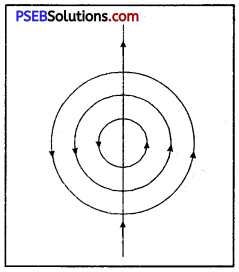(d) concentric circles centred on the wire (Figure)

Question 2.
The phenomenon of electromagnetic induction is :
(а) the process of charging a body.
(b) the process of generating magnetic field due to a current passing through a coil.
(c) producing induced current in a coil due to relative motion between a magnet and the coil.
(d) the process of rotating the coil of an electric motor.
(c) producing induced current in a coil due to relative motion between a magnet and the coil.

Question 3.
The device used for producing electric current is called :
(a) generator
(b) galvanometer
(c) ammeter
(d) motor.
(a) generator.

Question 4.
The essential difference between an AC generator and a DC generator is that:
(а) AC generator has an electromagnet while a DC generator has a permanent magnet.
(б) DC generator will generate higher voltage.
(c) AC generator will generate higher voltage.
(d) AC generator has slip rings while the DC generator has a commutator.
(d) AC generator has slip rings while DC generator has a commutator.

Question 5.
At the time of short circuit, the current in the circuit.
(a) reduces substantially
(b) does not change
(c) increases heavily
(d) vary continuously.
(c) increases heavily.

Question 6.
State whether the following statements are true or false.
(a) An electric motor converts mechanical energy into electric energy.
(b) An electric generator works on the principle of electromagnetic induction.
(c) The field at the centre of a long circular coil carrying current will be parallel straight lines.
(d) A wire with a green insulation is usually the live wire.
(a) is false. It converts electric energy to mechanical energy.
(b) is true.
(c) is true.
(d) is false. Green is usually earth wire.

Question 7.
List three sources of magnetic field.
Sources of Magnetic field are :

• Magnet.
• Current carrying conductor
• Current carrying solenoid.

Question 8.
How does a solenoid behave like a magnet? Can you determine north and south poles of current carrying solenoid with the help of bar magnet? Explain.
Solenoid: It consists of a coil of a number of turns of insulated copper wire closely wound in the shape of a cylinder. Magnetic field around a current carrying solenoid is shown in Figure.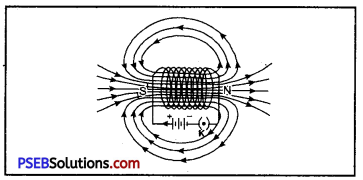Field lines of the magnetic field inside and around a current carrying solenoid.

These magnetic lines due to current carrying solenoid appear to be similar to that of a bar magnet shown in Figure.

One end [right end] of solenoid behaves like north pole and the other end [left end] behaves like south pole. Magnetic field lines inside the solenoid are in the form of parallel straight lines. This means that the field is the same at all points inside the solenoid.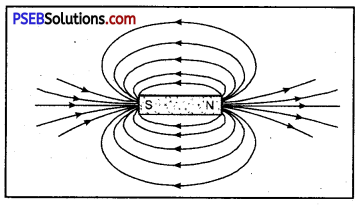Field lines around a bar magnet.

A soft iron rod when placed inside the solenoid behaves, like an electromagnet.

Question 9.
When is, the force experienced in a magnetic field, the largest?
When the field is perpendicular to current carrying conductor, the force experienced by a current carrying conductor placed in a magnetic field is largest.Question 10.
Imagine that you are sitting in a chamber with your back to one wall. An electron beam moving horizontally with back towards the front wall, is deflected by a strong magnetic field to your right side. What is the direction of the magnetic field?
The magnetic field will be acting in vertically downward direction in accordance with Fleming’s left hand rule. [Direction of the current should be considered in a direction opposite to the direction in which the electrons move].

Question 11.
Draw a labelled diagram of an electric motor. Explain its principle and working. What is the function of a split ring in electric motor?
Electric motor: It is a device which is used to convert electric energy into mechanical energy.
Principle, “When a current carrying coil is placed in a uniform magnetic field, it experiences a torque which rotates the coil.”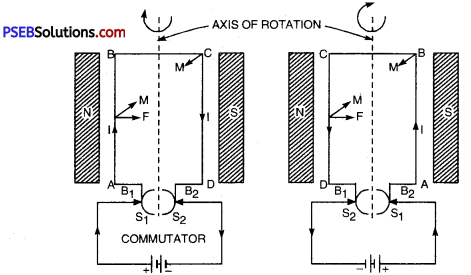I indicates direction of current; F the direction field and M the direction of motion

Working: A direct current from a battery is passed through armature. The current flows in the coil along ABCD as shown in Figure (a). The limb AB of the coil experience downward and CD of the coil experience upward force in accordance with Fleming’s left hand rule. These two equal and opposite forces constitute a couple tending to rotate the coil in clockwise direction. After half the rotation, brush B1 has contact with S2 and brush B2 with S1. The direction of the current gets reversed. The current now flows along DCBA instead of along ABCD. Limb DC experiences downward and BA experiences an upward force in accordance with Fleming’s left-hand rule.

The process repeats itself and motion of armature becomes continuous after some time.
Functions of Split rings. They help in reversing the direction of current in the coil after every half rotation.

Question 12.
Name some devices in which electric motors are used.
Electric motor are used in battery operated toys, in tape recorder, in car fans, mixers, grinders, computers and variety of other electric appliances.

Question 13.
A coil of insulated copper wire is connected to a galvanometer. What will happen if a bar magnet is (i) pushed into the coil; (ii) withdrawn from inside the coil; (iii) held stationary in the coil?

• When the bar is pushed into the coil, there will be momentary deflection of galvanometer in one direction. This is so because when magnet is brought near the coil, magnetic lines linked with the coil increases, so that induced emf is produced which induces current in the coil.
• Faster, we push the magnet, more will be the deflection.
• When the bar magnet is withdrawn, there will again be momentary galvanometer deflection but in a direction opposite to that when magnet was pushed in. This time also current is induced in the coil.
• When the magnet is held stationary inside the coil, there will be no deflection in galvanometer. It is because no emf is induced and hence no current is induced in the coil.

Question 14.
Two circular coils A and B placed closed to each other. If the current in the coil A is changed, will some current be induced in coil B? Give reason.
If the current in the coil is changed (switched on or switched off), then an electric current is induced in coil B. ‘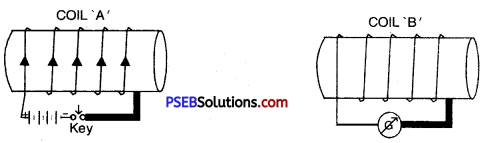It is because when, plug in the key is introduced, current flows through the coil ‘A’ so that magnetic field is produced all round it. These magnetic lines produced in the coil ‘A’ will pass through the coil ‘B’ with the result induced emf and hence induced current is produced in the coil ‘B’ which is indicated by deflection of the galvanometer. Now when plug is removed from the key (switched off) the magnetic lines of force Jinked with the coil ‘B’ again change (decreases). This time again current is induced in the coil ‘B’.

Question 15.
When does an electric short circuit occur?
Electric short-circuit occurs when :

• live wire incidently touches neutral or earth-wire.
• insulation around the current carrying wires is weak.
• insulation gets hardened by the excessive use.
• current passed through wire is more than its rating.

Question 16.
What is the function of earth wire? Why is it necessary to earth metallic appliances?
Earth wire. It is used as a safety measure especially for electric appliance having metallic body. The metallic body of appliances like electric press, fans, toasters, refrigerators etc. are connected to earth wire which provides an easy path for current to go to the earth in case live wire touches the body of appliance incidently. The user will not suffer a severe electric shock in the event of touching a defective appliance.

Question 17.
State two properties of magnetic field lines.

1. Magnetic field start from north and end at south.
2. They never intersect each other.

Science Guide for Class 10 PSEB Magnetic Effects of Electric Current InText Questions and Answers

Question 1.
Why does a compass needle get deflected when brought near a bar magnet?
A compass needle is a small bar magnet with one end as north and the other end as south pole. It is a well known fact that similar poles repel each other and dissimilar poles attract each other. When N-pole of a bar magnet is brought near the compass, the north pole of compass gets repelled while its S-pole is attracted so that compass needle gets deflected.

Question 2.
Draw magnetic lines around a bar magnet.
Place a bar magnet in the middle of a sheet of paper fixed on drawing board by using adhesive tape. Mark the boundary of the magnet with pencil. Place compass near north pole of the magnet when south pole of the compass will point towards north pole of the magnet. Mark the two points a, b at the two ends of the needle. Move the needle to a new position such that S-pole of compass needle is at b [position previously held by N-pole].

Repeat this till you reach south pole of the magnet (Figure).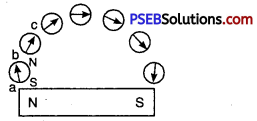Drawing magnetic field lines by compass needle.

Now Join the marked points on a paper by a smooth line which gives one magnetic line of force as shown in Figure. Repeat this procedure taking Figure Magnetic field around a bar different starting points.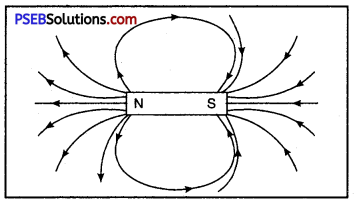Magnetic field around a bar magnet.Question 3.
List the properties of magnetic lines of force.
Or
Write characteristics of magnetic field lines.
Properties (characteristics) of magnetic lines of force are :

• These are the closed curves passing through the magnet. Outside the magnet lines of force start from north pole of the magnet and end at the south pole and inside the magnet, the direction of magnetic lines are from south pole to north pole.
• The two magnetic lines of force never intersect each other.
• They have a tendency to contract lengthwise which explains the attraction between opposite poles.
• The tangent at any point of the magnetic lines of force gives the direction of the field at that point.
• They exert lateral pressure upon each other, which explains repulsion between like poles.

Question 4.
Why two magnetic lines of forces never intersect each other?
No two magnetic lines of force cross each other because, if they did so, then at the point of intersection; the compass needle would point towards two directions at the same time, which is not possible. Hence two magnetic lines never intersect each other.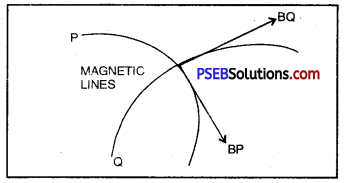Question 5.
Consider a circular loop of wire lying in the plane of the table. Let the current pass through the loop clockwise. Apply right hand rule to find out the direction of the magnetic field inside and outside the loop.
The direction of the magnetic field inside and outside loop is as shown in Figure. By applying right hand rule we find that the direction of magnetic field inside the loop is downward normally and outside the loop it is normal to the plane of paper.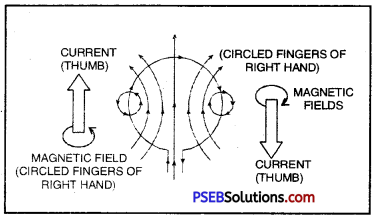Question 6.
The magnetic field in a given region is uniform. Draw a diagram to represent it.
Uniform magnetic field is shown by equidistant and parallel lines as shown in Figure If the parallel lines are close to each other, the field is strong. The stronger the field, the closer are the lines.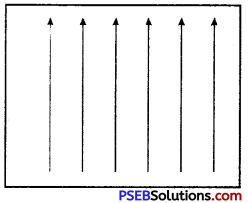Question 7.
Choose the correct option.
The magnetic field inside a long straight solenoid carrying current (a) is zero ;
(b) decreases as we move towards end ;
(c) increases as we move towards end;
(d) is same at all the points.
(d) is correct. The magnetic field inside a long straight solenoid is same at all the points.

Question 8.
Which of the following property of proton can change while it moves freely in a magnetic field? [There may be more than one correct answer].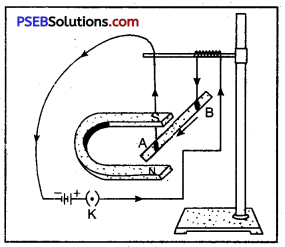(a) mass
(b) speed
(c) velocity
(d) momentum.
(c) Velocity and (d) Momentum.

Question 9.
In activity shown, how do you think the displacement of rod AB will be affected :
(i) if the current in rod AB is increased;
Since force acting on the rod is directly proportional to the current passing through it. Therefore, the displacement will be increased when current is increased.

(ii) a stronger horse shoe magnet is used;
Stronger the magnet, more will be the force and hence the displacement.

(iii) length of the rod AB is increased.
Force is also directly proportional to the length of the rod. Hence rod will be displaced more if the length is increased.

Question 10.
A positively charged particle emitted from a nucleus alpha particle projected towards west is deflected towards north by a magnetic field. The direction of the magnetic field is :
(a) towards south
(b) towards east
(c) downward
(d) upward.
(d) upward [In accordance with Fleming’s left hand rule]

Question 11.
State Fleming’s left hand rule.
Fleming’s left hand rule. It states, “Stretch the thumb, fore finger and middle finger of your left hand such that they are mutually perpendicular to each other. If the first finger points in the direction of magnetic field and central (second) finger points towards the direction of current then thumb points towards the direction of motion as shown in Figure.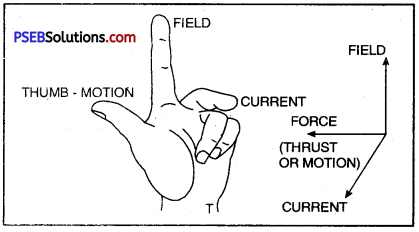Flemmgs left hand rule for direction of force on current carrying conductor

Question 12.
What is the principle of an electric motor?
Principle of Electric motor. Electric motor is based upon the principle that when a current carrying coil is placed in a uniform magnetic field, it experiences torque which rotates the coil.

Question 13.
What is the role of the split ring in an electric motor?
The split rings act as a commutator in D.C. motor i.e., it reverses the direction of current through the circuit after every half cycle.Question 14.
Explain different ways to induce current in a coil.
There are mainly two methods to induce current in a coil.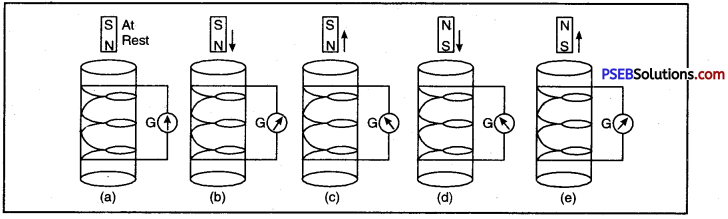Induced current due to relative motion between coil and magnet.

When the magnet is stationary [at rest as in Figure (a)], no induced current is produced.
1. When magnet is moving with its N-pole towards the coil, deflection in galvanometer is as shown in Figure (b). Direction of the current gets reversed when N-pole is withdrawn from inside the coil (going away from coil) as shown in Figure (c). Faster the magnet moves, more is the deflection and hence current in galvanometer.

Deflection produced in the galvanometer needle by the current is same when N-pole was moving down [Figure (6)] or when south pole is out of the coil [Figure (e)].

Current and hence deflection in the galvanometer needle will be again produced if magnet is kept at rest and coil is moved.

2. By changing current in the neighbouring circuit. Take a non¬conducting cylindrical tube (say of card board). Wind two set of coils I and. II on it as shown. Connect a battery and a key to the ends of coil I and a galvanometer to the ends of coil II. When plug is inserted in key K, there will be an instantaneous deflection m galvanometer even though there is no cell in this circuit. Now take out the plug from key K. An instantaneous large deflection in opposite direction to previous deflection will be produced in galvanometer. Thus current has been induced in coil II due to increase or decrease of current in coil I.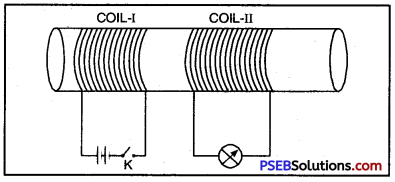Induced Current by changing current m the neighbouring circuit.

Question 15.
State the principle of electric generator.
Principle of Electric Gen¬erator. Electric generator is based upon Fleming’s right hand rule.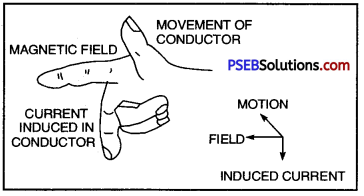Right hand rule

Fleming’s right hand rule. Stretch the thumb, fore-finger, control (middle) finger of right hand so that they are perpendicular to each other. If fore-finger indicates the direction of magnetic field, the thumb shows the direction of motion of the conductor, the central (middle) finger will show the direction of induced current.

Question 16.
Name some sources of direct current.
Sources of direct current are

• Cell
• Battery
• D.C. generator.

Question 17.
Which sources produce alternating current?
Alternating current is produced by A.C. generators. There are hydro-generator and thermal generators.

Question 18.
Choose the correct option :
A rectangular coil of copper wires is rotated in magnetic field. The direction of induced current changes once in each:
(a) two revolutions
(b) one revolution
(c) half revolution
(d) one-fourth revolution.
(c) half rotation.

Question 19.
Name two safety measures commonly used in electric circuits and appliances.
Or
Write the two safety measures commonly used in electric circuit appliances.
Common Safety Measures used in Electric Circuits. Two most common safety measures used, are :

• A safety fuse of proper rating connected in a circuit prevents damage to the electric appliances and also the circuit due to overloading or short circuiting.
• Earth wire prevents possible electric shock when live wire incidently touches the body of appliance.

Question 20.
An electric oven of 2kW power rating is operated in a domestic electric circuit (220 V) that has a current rating of 5 A. What result do you expect? Explain.
Given : Power of oven (P) = 2 kW
= 2,000 W
Voltage (V) =220 volts
Current (I) =?

We know that, P = V x I
or I = $$\frac{\mathrm{P}}{\mathrm{V}}$$
Current (I) = $$\frac{2,000}{200}$$
= $$\frac{100}{11}$$
= 9.09 A

A current of 9.09 A will flow in the circuit. Since the current rating of circuit is 5 A, the fuse (of 5 A) rating if inserted in the circuit will burn up. If no fuse is placed in the circuit, there may be a fire.Question 21.
What precautions should be taken to avoid the overloading of domestic electric circuits?